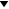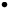# PHYSICS

## Notes about these Notes

There is only one section available here.

(14/02/10) Hey bro, you’ve been here a fair bit over the last month. Hope you aren’t plagiarising ;)

# 9.2 Space1 The Earth has a gravitational field that exerts a force on objects both on and around itDefine weight as the force on an object due to a gravitational field
 The gravitational field around an object exerts a force on all other objects. This force is called weight.Explain that a change in gravitational potential energy is related to work done
 Work is Force times distance. Work is also mass times gravity times height which also happens to be gravitational potential energy. Therefore Work is related to gravitational potential energy.Define gravitational potential energy as the work done to move an object from a very large distance away to a point in a gravitational field
 See the formula.2 Many factors have to be taken into account to achieve a successful rocket launch, maintain a stable orbit and return to EarthDescribe the trajectory of an object undergoing projectile motion within the Earth's gravitational field in terms of horizontal and vertical components
 Tip: When calculating motion it is good to break the motion into horizontal and vertical components.Describe Galileo's analysis of projectile motion
 Experimented with balls on ramps. Found:1. Final velocity and acceleration were independent of mass. That is, they did not affect each other2. Projectile motion should be in the shape of a parabola.Explain the concept of escape velocity in terms of the: gravitational constant; mass and radius of the planet
 G is the gravitational constant. M is the mass of the planet. R is the radius of the planet IF you are on the surface.Outline Newton's concept of escape velocity
 Escape velocity is how fast you need to be going at a point to escape the planet's gravitational field. If a cannon on a high mountain gave enough kinetic energy to a ball, it would go into orbit. If it gave more, the ball would spiral away from earth.Identify why the term 'g forces' is used to explain the forces acting on an astronaut during launch
 G Forces are used to describe the amount of force an astronaut is feeling. This is in relation to gravity of Earth. G Forces describe the amount of gravity acceleration the person is feelingDiscuss the effect of the Earth's orbital motion and its rotational motion on the launch of a rocket.
 A point on the equator is moving at 460 ms-1 towards the east. Therefore it is best to launch into the east to avoid trying to orbit against the rotation of the Earth.Analyse the changing acceleration of a rocket during launch in terms of the:Law of Conservation of Momentum
 If a rocket is launched to the east on the equator, the velocity of the earth will help to put the shuttle into orbit. Because the surface is already going at 460 m/s at the equator, the rocket must also be so its momentum is conserved.forces experienced by astronauts
 The rocket slowly accelerates as it launches. At first the acceleration is small as it fights with the gravity of the earth but as its mass decreases and g decreases as it gets further away, the force on the astronauts increases as they move faster.Analyse the forces involved in uniform circular motion for a range of objects, including satellites orbiting the Earth
 A thing moves in a circle if it is moving with centripetal motion. The thing's speed doesn't change but it's direction does. If you combine the centripetal force and gravitational force equations (F=F) you can get the equation to find the velocity of a satellite in orbit at a certain distance with a certain mass (its almost the escape velocity formula). They can orbit in ellipses.Compare qualitatively low Earth and geo-stationary orbits
 Low earth orbits:Closer to earth (a few hundred kilometres above the surface)Faster orbit time or periodFor telecommunications satellites Their orbit can decay due to earths atmosphere resistanceGeostationary orbits:Further from earth (36000km)Period of one dayGPS SatellitesNo dragDefine the term orbital velocity and the quantitative and qualitative relationship between orbital velocity, the gravitational constant, mass of the satellite and the radius of the orbit using Kepler's Law of Periods
 There is an equation that they give you in the HSC. Orbital velocity is how fast a satellite travels in a circle.Account for the orbital decay of satellites in low earth orbit
 Satellites in low earth orbit encounter the resistance of the atmosphere while orbiting. The constant bombardment by particles slows down the satellite over time.Discuss issues associated with safe re-entry into the earth's atmosphere and landing on the Earth's surface
 Heaps of things can go wrong if you re-enter the atmosphere wrong. If you enter the atmosphere too quickly at a steep angle, the ship could burn up from friction. The fast deceleration could also damage the crew members. If the angle is not steep enough it could bounce off the atmosphere back into space. An angle of 5 to 7 degrees is good as long as the ship is not going too fast and it has enough insulation. You could also use braking ellipsis where it comes back around after bouncing off and keeps bouncing until it looses enough energy.Identify that there is an optimum angle for safe re-entry for a manned spacecraft into the Earth's atmosphere and the consequences of failing to achieve this angle
 If you enter the atmosphere too quickly at a steep angle, the ship could burn up from friction. The fast deceleration could also damage the crew members. If the angle is not steep enough it could bounce off the atmosphere back into space. An angle of 5 to 7 degrees is optimum as long as the ship is not going too fast and it has enough insulation.3 The Solar System is held together by gravityDescribe a gravitational field in the region surrounding a massive object in terms of its effect on other masses in it
 The closer that an object gets to a massive object, the more force gravity exerts on it.Define Newton's Law of Universal Gravitation
 Force between two objects is the product of the mass of the two objects divided by the distance between them squared times the Gravitational constant.Discuss the importance of Newton's Law of Universal Gravitation in the understanding and calculating the motion of satellites
 When we use the equation and make it equal to the centripetal force equation we can derive the escape velocity formula. This is useful to help scientists calculate the fuel and forces needed to launch a rocket and keep it in orbit.Identify that a slingshot effect can be provided by planets for space probes
 The slingshot effect is used to send space probes into space faster while using less fuel. This works by changing the probe to a hyperbolic orbit: the probe is swung around one side of the planet until it has enough momentum to break out of orbit and into space. Due to the law of conservation of momentum, this means that the kinetic energy of the planet is transfered to the probe.4 Current and emerging understanding about time and space has been dependent upon earlier models of the transmission of lightOutline the features of the aether model for the transmission of light
 Back in the day (19th C)people thought that light travelled through a medium called the aether because all waves travelled through a medium. The aether penetrated all mater and was permeated by all matter. It filled all of space and was invisible. It was stationary compared to all bodies.Describe and evaluate the Michelson-Morley attempt to measure the relative velocity of the earth through the aether
 If the aether is stationary, the Earth moves through it, and light uses it to travel, then light on earth should be faster in one direction than in ninety degrees to that direction because it would be fighting against the aether in much the same way that a swimmer fights the current in a river. The river is the aether: one swimmer swims across it while another swimmer swims up stream. They will have different swim times if they swim the same distance. This was the reasoning behind the experiment.A light was split into two beams and sent in two directions perpendicular to each other. They return to a single point having travelled the exact same distance. Here they interfere with each other like all good waves do. If the apparatus of this experiment was turned in different directions, the interference patters seen should change. They didn't, which proved that the earth was not moving through an aether: there was no aether.Discuss the role of the Michelson-Morely experiments in making determinations about competing theories
 There were two theories: aether or no aether. The experiment was designed to measure the speed that the Earth moves through the aether but they got a null result. This proved the no aether theory. It determined once and for all that that theory was correct.Outline the nature of inertial frames of reference
 An inertial frame of reference is one where Newton's first law applies, that is, a frame that moves with constant or no velocity relative to another frame.Discuss the principal of relativity
 If the speed of light is constant from all frames of reference then the measurements of time and length are different and relative to the speed of that frame of reference.Describe the significance of Einstein's assumption of the constancy of the speed of light
 Einstein just assumed that light always moved at a constant speed relative to everything. This would contradict Galileo's observations of movement in different frames but he decided that for both of them to be true, length and time must change in each frame.Identify that if c is constant then space and time must become relative
 If the speed of light is constant then time and length become variables in equations to make sure that they still work.Discuss the concept that length standards are defined in terms of time in contrast to the original metre standard.
 The metre used to be the length of a certain metallic bar. However, when it was discovered that c is a constant, a more accurate measurement of the meter was obtained because the length of the bar might have contracted due to different frames of reference.Explain qualitatively and quantitatively the consequence of special relativity in relation to:the relativity of simultaneity
 Simultaneous event in one frame may not be simultaneous in another frame.the equivalence between mass and energy
 E = mc2If space and time are related then mass and energy must be too.length contraction
 As something approaches the speed of light, its length contracts to zero in the direction of movement.time dilation
 As something approaches the speed of light, time slows downmass dilation
 As an object approaches the speed of light, it's mass becomes infinite.Discuss the implications of mass increase, time dilation and length contraction for space travel
 Speed of light travel is impossible because you would have an infinite mass which requires an infinite force to move.Time would slow down as you approach the speed of light so people could live to stand on distant planets if they travelled fast enough. You would squish down if you went very fast.